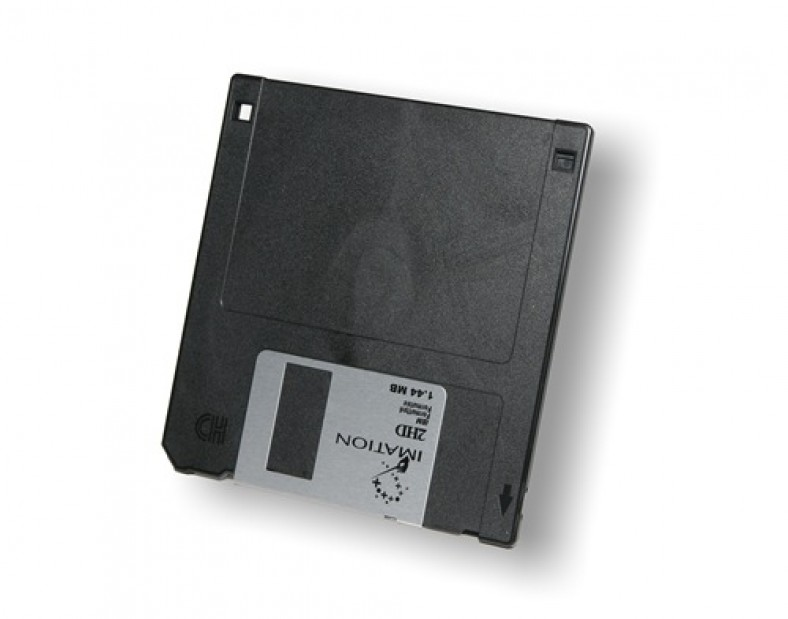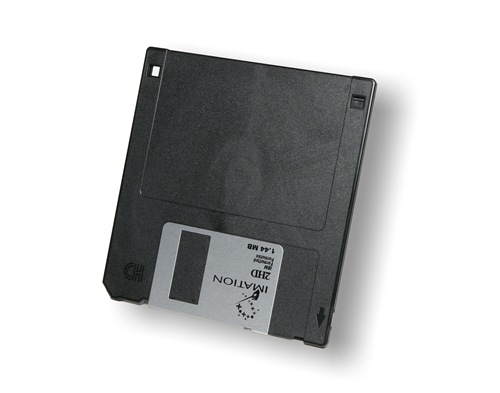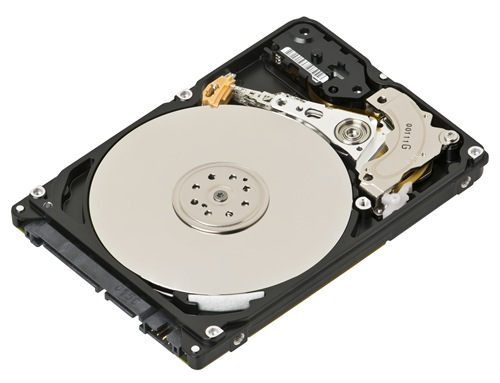# How many kilobytes in a megabyte or gigabyte

How many kilobytes in a megabyte? There are 1024 kilobytes is equal to a megabyte.

Related articles about How many kilobytes in a megabyte

How many MB (megabytes) in a GB

How many stamps do I need?

How many calories in an egg

A byte is a used for the measurement of digital information. It is made of 8 bits. The byte is used n C and C++ programming. The byte is a unit of data. It is used to demonstrate any letter or number such as 5, 7, x, y and z. In the processor structures, the four bytes establish a word. A byte is written as “B” and bit is written as “b”. You probably hear about kilobytes and megabytes every day. It is an important part of our mobile phones and PC computers.1024 kilobytes is actually 1 megabyte but they have made it into 1000 Kilobytes for ease. The kilobytes are a bigger unit that bytes and is written as KB. The megabyte is a bigger unit which is written as MB. The terms related to bytes are: bits, bytes, kilobits, kilobytes, megabits, megabytes, gigabits, gigabytes, terabytes and petabytes.

A kilobyte is 1024 bytes; hence you need to multiply 1024 with 8 which is 8192 digits. A megabyte is equal to 1024 KB. A kilobyte is a unit of data computer storage equal to 1024 bytes, supposing a binary system. A megabyte is a unit of data which is equal to 1,048,576 bytes.

Calculation: 1024 bytes = 1 KB

1048576 bytes = 1 MB

How many kilobytes in a gigabyteThere 1,048,576 Kilobytes in a gigabyte

People have questions like whether 1 GB is equal to 1024 MB or 1000 MB. It actually varies because hard drives those are publishes in gigabytes are 10 to the power 9 bytes and the OS consider gigabytes using 2 to the power 30 bytes. Therefore, 1 GB in published size is 1,000,000,000 bytes. 1MB is 1,024 multiplied by 1,024 bytes which ends in 1GB being equal to 953MB. 1MB in hard drive publicized size is 1,000,000 bytes therefore 1KB is 1,024 bytes so 1MB is 976KB.

If you are talking about the sizes in your OS you will say that 1 GB is 1024 MB, 1 MB is 1024 KB and 1 KB is 1024 bytes. Therefore, a 210 GB hard drive has 210, 000, 000, 000 bytes. In short you need to know that other storage formats like HDD, DVD, flash drives use the KB, GB and MB. There they use 1 KB = 1000 bytes, 1 MB = 1000 KB and 1 GB = 1000 MB.

To find out how many kilobytes are in a gigabyte, you need to remember the easy method. It is just multiplying 1024 with 1024.

Calculation:

1 GB = 1024 MB

1 MB = 1024 KB

1 KB = 1024 bytes

Therefore, 1 GB = 1024 X 1024 KB